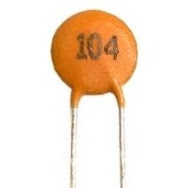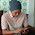# How to read, or calculating capacitor value?

Capacitance value is often printed on the capacitor body, because there is not enough space on bodies of small-sized capacitors, the capacitance values ​​must be encoded.Code that is used consists of three digits. The first two digits of code of is the first two digits of the value of the capacitor is concerned, in units pikofarad (pF). The third digit represents the number of zeros contained in the back of two digits earlier.

Example question 1:
What is the value of capacitance of capacitors shown in the picture above?

completion:
The capacitor has a code 104, meaning that 10 followed by 4 pieces of zeros behind it. By using the value conversion units, then
C = 100000 pF
C = 100 nF
C = 0.1 μF
Capacitor values ​​obtained in the image above is 0.1 μF

Example question 2:
How much the value of the capacitor with code 223, 122 and 471?

completion:
1. The code 223 means that 22 followed by 3 pieces of zeros behind it. This value are 22 000 pF, equal to 22 nF
2. The code 122 means that 12 followed by 2 pieces of zeros behind it. This value is 1200 pF, equal to 1.2 nF
3. The code 471 means that 47 is followed by 1 piece of zero behind it. This value are 470 pF, equal to 0.47 nF

Next→
←Prev

### Choose label

1.whate men 47/ML printed a capasiter

2.a capasiter printed only two digit printed
"47" how to clculats it's value

3.capacitor has a code 47, it's mean the value of capacitor is 47 pF (pikofarad, 10^-6 Farad) :h:

4.5.At that point the plates remain charge impartial as an expected contrast because of this charge is set up between the two plates. The measure of potential contrast present across the capacitor relies on how much charge was saved onto the plates by the work being finished by the source voltage and furthermore by how much capacitance the capacitor has. high voltage ceramic capacitor

6.There are a wide range of sorts of capacitors accessible from tiny capacitor globules utilized in reverberation circuits to huge force factor amendment capacitors, however they all do exactly the same thing, they store charge.
China Power Mosfet manufacturer

7.I never knew that Capacitance value is often printed on the capacitor body, because there is not enough space on bodies of small-sized capacitors as I always used matrix calculator for calculation.

8.You can check in without spending a dime to store and percentage your graphs, that is notable, for the reason that maximum on-line college students are required to put up digital graphs nowadays, and maximum on-line calculators do not provide you with this choice.click site